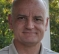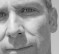## Singularity issue due to Zero-frequency componentStarted by 4 years ago4 replieslatest reply 4 years ago89 views

I am a Ph.D. student working on nonlinear elastic modeling of elastic materials in the Netherlands. In my formulation, I have a singularity issue at the zero frequency component in the expression of force potential function $$\mathrm{b}(k,t)$$, as follows.

$$f(x,t)= Q\: \cos(\omega \:t)\:\delta(x)$$

where, $$f(x,t)$$ is the given input force function,

$$Q$$ is the input amplitude

$$\cos(\omega \:t)$$ is the sine signature pulse

$$\delta(x)$$ is the delta pulse acting at $$x=0$$

The fource (vector) function is related to the scalar potential as follows:

$$f(x,t)= \frac{\mathrm{db}(x,t)}{\mathrm{d}x}$$

I need to compute the potential function $$b(x,t)$$, by transforming the above expression in frequency domain, which becomes,

$$\tilde{f}(k,t)=-\mathrm{j\:k} \:\tilde{\mathrm{b}}(k,t)$$

$$\tilde{\mathrm{b}}(k,t)=\frac{\mathrm{j}}{\mathrm{k}} \:\tilde{\mathrm{f}}(k,t)$$

where $$k$$ is the angular spatial frequency, $$k=2\:\pi \:(0:M-1)\:dk$$, $$M$$ is the number of spatial points and $$dk$$ is the spatial frequency interval. When I implement the expression  $$\tilde{\mathrm{b}}(k,t)$$ in Matlab, I get the infinity value as I have zero frequency component in $$k$$ array (the first value of $$k=0)$$.

I tried to eliminate the singularity by doing the Taylors series expansion of $$\tilde{\mathrm{f}}(k,t)$$, but it wasn't useful, as I have $$\mathrm{rect(k)}$$ rectangular function in the expression for $$\tilde{\mathrm{f}}(k,t)$$.

I simply replace the invalid infinite value to zeros in the expression for $$\tilde{\mathrm{b}}(k,t)$$, which I justify as follows: At zero frequency value, the signal starts to oscillate at the constant frequency, whose function value at zero frequency can be set to zero, which I did in $$\tilde{\mathrm{b}}(k,t)$$.

Is this correct? If not, how can I fix this infinity issue?. Any ideas/advice would be highly appreciable.

[ - ]Sorry to say I don't have an answer, but rather a question to consider.

From a practical point of view, can your forcing function, applied to your non-linear elastic material, have a "DC" component?  If so, then eliminating the zero frequency case should not be valid.

I don't know much about non-linear elastics, but it seems to me that a "DC" forcing function will have a non-zero effect (at other frequencies) on the material, especially since it is non-linear.  Just a guess.

[ - ]Hi,

if you want your equation to be displayed inline, use:

$$e^{i\pi}+1=0$$


$$e^{i\pi}+1=0$$

[ - ]